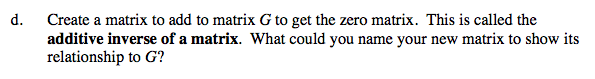Home > A2C > Chapter 7 > Lesson 7.3.1 > Problem7-155

7-155.
1. Let G be the matrix shown below. Homework Help ✎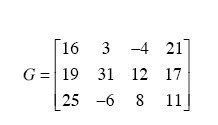1. What is g1,3

2. Two matrices can be added only if they have the same dimensions. If matrix H can be added to matrix G, what must be the dimensions of H?

3. All the entries of the zero matrix are 0. Write the zero matrix with the same dimensions as G.

4. Create a matrix to add to matrix G to get the zero matrix. This is called the additive inverse of a matrix. What could you name your new matrix to show its relationship to G?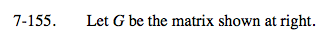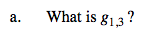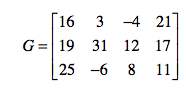g3,2 is the entry in the 3rd row and 2nd column. For the given matrix, g3,2 is −6.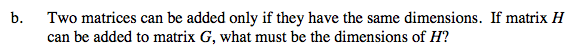See part (a).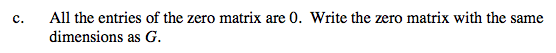See part (a).